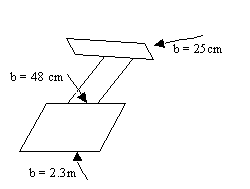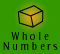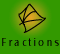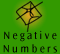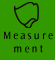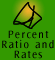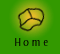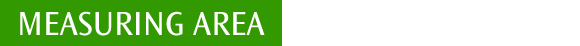| Measuring Area | Area measurement facts |
| Summary of area formulae of some shapes| Quick quiz |

Measuring Area

Area is the amount of surface of an object. The area of regular, irregular and complex plane figures can be measured. Both flat and curved surfaces have area.

Example 1: Work out the area of the coloured region if the coloured square is the unit.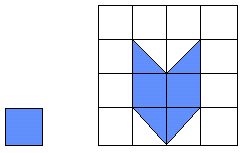If we slide the two blue triangles in the bottom row of the grid up to the second row of the grid we can see that in total four units on the grid are coloured blue, so the area is 4 times the area of the coloured square.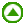Area measurement facts

AREA SI unit: square metre (m2)
100 square millimetres (mm2) = 1 square centimetre (cm2)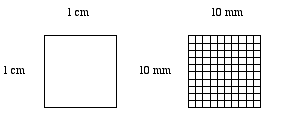1 cm2 = 1 cm x 1cm = 10 mm x 10 mm = 100 mm2

NOTE: The illustration has been enlarged,
1 cm is actually smaller than pictured above

10 000 square centimetres (cm2) = 1 square metre (m2)
10 000 square metres (m2) = 1 hectare (ha)
100 hectares (ha) = 1 square kilometre (km2)

 Odd spot: An are is the basic unit for measuring area. A hectare, our common unit for measurement of area of land, is 100 ares. It is the area of a square 100m by 100m, but of course it can be any shape.Summary of area formulae of some shapes:

 Area of a square = l x l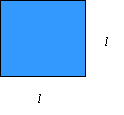Area of a rectangle = l x w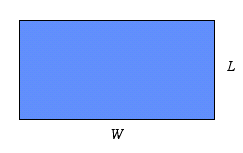Example 2: A rectangular block of land is measured 300 m long and 120 m wide. Calculate the area of the block and express your answer in hectares.

300 m

120 m

 Setting Out Thinking Area = Length x Width = 300 m x 120 m = 36000 m2 = 3.6 hectares The length is 300 m and the width is 200 m, so I need to multiply these measurements together. Metres x metres is square metres, so the units of my answer are square metres. I know that 10000 m2 = 1 hectare and hectares are larger than square metres so I divide by 10000. So, 36000 m2 = 3.6 hectares.

Example 3: Movie - intermediate explanation of calculation of the area of a rectangular object

 In this movie the narrator has divided the object into 2 rectangles. Can you see another way to divide this object and calculate its area?

Area of a triangle = 1/2 x (b x h) where b is length of base and h is the height measured perpendicular to the base.

We can show justification for the formula of the area of a triangle by looking at the area of a square.

 Justification for the formula of the area of a triangle Thinking Text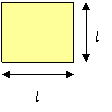Area of a square = l x l We know the area of a square can be found by multiplying the length of 2 sides (l). Area of a square = l x l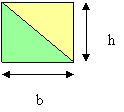Area of a square = b x h If we divide a square in half diagonally we can see that the square has the same area as the 2 triangles. We can rename the sides of the square as base (b) and height (h) and the area of the square can also be expressed as, area = b x h. All we have done is use different labels for the sides of the square.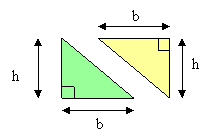Area of a triangle = 1/2 x b x h We can now see that the area of each equal sized triangle must be half of b x h because the area of the 2 triangles is b x h. That is, 1/2 x b x h. NOTE: We are referring to the height perpendicular to the base. This is called the perpendicular height. As these triangles are 'right-angled' triangles, the perpendicular height can easily be seen. It is not always as obvious as is shown in the following examples.

 The orientation of the triangle does not determine how the height is measured. The height of a triangle is always perpendicular to the base. The base can be any side. The perpendicular height connects from a vertex to the opposite side (which can be the base). It is measured at right angles to the chosen base.Example 4: Movie - full explanation of calculation of the area of a triangular shape.

What is the area of a triangular sheet of metal which has a perpendicular height of 1.2 m and a base of 3.7 m?

 Area of a parallelogram = b x h = length of base x height measured perpendicular to base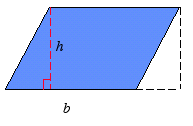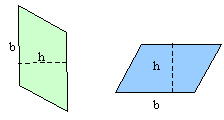The perpendicular height of a parallelogram is always measured at right angles to the chosen base, irrespective of the orientation of the parallelogram. The area formula works because the translation (a slide) preserves size and shape.

Example 5: Movie - finding the area of a parallelogram with a base of 8 cm and a perpendicular height of 4cm.

 Area of a trapezium = 1/2 x (a + b) x h = half sum of parallel side lengths x perpendicular height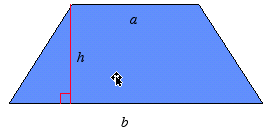The diagram below shows that 2 congruent trapeziums have been put together by flipping and sliding to form a parallelogram with a base of (a+b). So we can see that the the area of a trapezium can be found by finding the area of the resulting parallelogram, using (a+b) x h, and dividing it in half.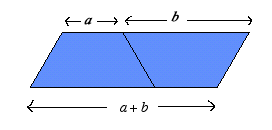We can say, in other words, the area of a trapezium is half the sum of the lengths of the parallel sides multiplied by the perpendicular distance between them.

 Area of a circle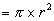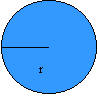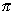, pronounced as 'pi', is a special number which is approximately equal to 3.142. As pi is used regularly in calculations of circular measurement most calculators will have abutton. 'r' is the radius of the circle, which is the distance from the centre of the circle to the edge of the circle. The diameter of a circle, 'D', is the distance across the circle through the centre. The diameter is twice the length of the radius. Can you express the area of a circle in terms of pi and its diameter, D?

 Justification for the area of a circle formulaBy dividing a circle into segments and then lining up each segment to form a parallelogram we can see an informal justification for the area formula for a circle. We know that the area of a parallelogram = base x perpendicular height, so using the picture above we can see that if we had taken even smaller segments of the circle and lined them up to form a parallelogram, the radius would eventually approximate the perpendicular height. The base of the parallelogram is formed by the outer edge of half the segments of the circle which is half the circumference of the circle. So the area of the 'parallelogram' can be approximated byx r2

Example 7: Movie - expert performance.

Calculation of the approximate area of a compound shape.Click on the image to view the movie Do you notice anything wrong with this movie? The narrator refers to the units of the shape as 'metres squared'. The correct expression is 'square metres'.Quick quiz

 1. Find the area of the following shapes: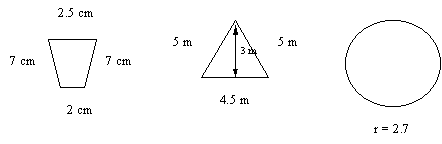2. Find the area covered by a circle with a radius of 4.5 cm. 3. Find the area of a parallelogram if its base is 12 cm and its perpendicular height is 3.4 cm. 4. I need to find the area of a kitchen bench which is rectangular in shape but which also has a circular breakfast area at one end. The actual shape of the bench is similar to a rectangle with a circle at one end. If the distance from one edge of the circular area to the centre of the circular area is 80 cm and the rectangular section of the bench is 2.3m long and 1 m wide. What is the approximate total area of the bench in metres? 5. Stanley needs to move some trapezoidal desks (which have a table top of the following dimensions) into a rectangular room which is 8m2 in area.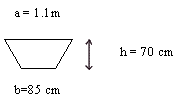Will he be able to fit the 12 of these desks comfortably into the room? 6. A pattern which is made up of parallelograms has been incorporated into the pavement paving and now needs to be covered with a non-slip coating. If the perpendicular height of each of the 3 parallelograms is the same, 1.8 metres, what area in square metres will need to be covered with the non-slip coating?